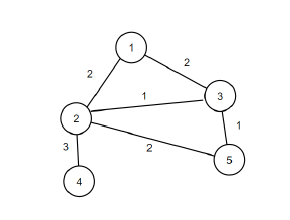# C++ Program to find out the number of bridge edges in a given graph

Suppose, we are given an unweighted, undirected graph that contains n vertices and m edges. A bridge edge in a graph is an edge whose removal causes the graph to be disconnected. We have to find out the number of such graphs in a given graph. The graph does not contain parallel edges or self-loops.

So, if the input is like n = 5, m = 6, edges = {{1, 2}, {1, 3}, {2, 3}, {2, 4}, {2, 5}, {3, 5}}, then the output will be 1.The graph contains only one bridge edge that is {2, 4}.

To solve this, we will follow these steps −

mSize := 100
Define an array G of size mSize
Define one 2D array bridge
Define an array visitedof size mSize
Define an array vk and l of size mSize
Define an array edges containing integer pairs
Define a function depthSearch(), this will take v, p initialize it with -1,
visited[v] := 1
vk[v] := (increase l[v] = t by 1)
for each x in G[v], do:
if x is same as p, then:
if visited[x] is non-zero, then:
l[v] := minimum of l[v] and vk[x]
Otherwise
depthSearch(x, v)
l[v] := minimum of l[v] and l[x]
if l[x] > vk[v], then:
bridge[v, x] := 1
Define a function bridgeSearch()
t := 0
for initialize i := 1, when i <= n, update (increase i by 1), do:
if not visited[i] is non-zero, then:
depthSearch(i)
for initialize i := 0, when i < m, update (increase i by 1), do:
a := first value of edges[i]
b := second value of edges[i]
insert b at the end of G[a]
insert a at the end of G[b]
bridgeSearch()
ans := 0
for initialize i := 1, when i <= n, update (increase i by 1), do:
for initialize j := 1, when j >= n, update (increase j by 1), do:
if i is not equal to j and bridge[i, j], then:
(increase ans by 1)
return ans

## Example

Let us see the following implementation to get better understanding −

#include <bits/stdc++.h>
using namespace std;

const int mSize = 100;
vector <int> G[mSize];
int n, m, t;
vector<vector<int>> bridge(mSize, vector<int>(mSize));
vector <int> visited(mSize);
vector <int> vk(mSize, -1), l(mSize, -1);
vector<pair<int, int>> edges;
void depthSearch(int v, int p = -1) {
visited[v] = 1;
vk[v] = l[v] = t++;
for (auto x: G[v]) {
if (x == p) {
continue;
}
if (visited[x]) {
l[v] = min(l[v], vk[x]);
} else {
depthSearch(x, v);
l[v] = min(l[v], l[x]);
if (l[x] > vk[v]) {
bridge[v][x] = 1;
}
}
}
}
void bridgeSearch() {
t = 0;
for (int i = 1; i <= n; ++i) {
if (!visited[i]) {
depthSearch(i);
}
}
}
int solve(){
for (int i = 0; i < m; ++i) {
int a, b; a = edges[i].first;
b = edges[i].second;
G[a].push_back(b);
G[b].push_back(a);
}
bridgeSearch();
int ans = 0;
for (int i = 1; i <= n; ++i) {
for (int j = 1; j <= n; ++j) {
if (i != j and bridge[i][j]) ans++;
}
}
return ans;
}
int main() {
n = 5, m = 6;
edges = {{1, 2}, {1, 3}, {2, 3}, {2, 4}, {2, 5}, {3, 5}};
cout<< solve();
return 0;
}

## Input

5, 6, {{1, 2}, {1, 3}, {2, 3}, {2, 4}, {2, 5}, {3, 5}}


## Output

1